# 2011 A-level H2 Mathematics (9740) Paper 1 Question 6 Suggested SolutionsAll solutions here are SUGGESTED. Mr. Teng will hold no liability for any errors. Comments are entirely personal opinions.

(i)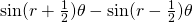(ii)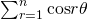(iii)

Let P(n) be the statement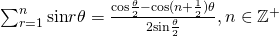When,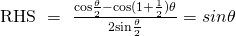Assume P(k) is true for some positive integers k,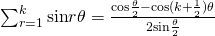To prove P(k+1) is true,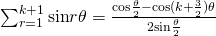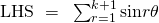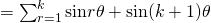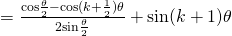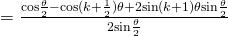, P(k+1) is true.

Since P(1) is true, and P(k) is trueP(k+1) is true, by mathematical induction, P(n) is true for all positive integers n.

Not readable? Change text.